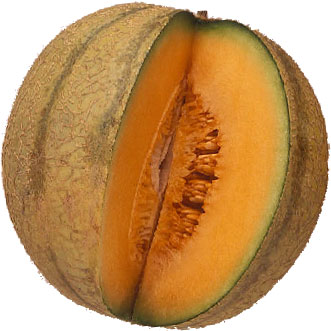SEARCH HOMEMath Central Quandaries & QueriesQuestion from Jaroslaw: Is there a formula or method to divide the surface area of a sphere into equal parts. For example; 36 equal parts, 60 equal parts or 92 equal parts and so forth.Jaroslaw,

If you wanted to divide a melon into equal sized parts - any number of
them - how would you slice it up?Cheers,
Stephen La Rocque.

Jaroslaw wrote back

My first question did not pose the problem adequately. Is there a method/formula one can use to place 36, 60 or 92 points, all equally distant from each other? This was the problem facing the Los Alamos scientists in 1944 and 1945.

There is still a problem with the question: you can have at most 4 points on a sphere that are equidistant from one another. You perhaps want each of n given points to be arranged on the sphere to be equidistant from some fixed number, perhaps 3, of the other points of the set. Another typical problem is to arrange the n points so that the average distance is as small as possible, or that the pattern is as regular as possible. Or perhaps you want a 2-distance set, or a 3-distance set. Anyway, I am not familiar with the problem (that you referred to) facing the Los Alamos scientists.

Chris

Jaroslaw,

There is a real treasure trove of analyses here:
http://www.math.niu.edu/~rusin/known-math/index/spheres.html

Cheers,
Stephen.Math Central is supported by the University of Regina and The Pacific Institute for the Mathematical Sciences.